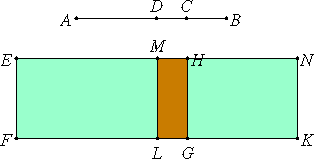# Proposition 47

The side of the sum of two medial areas is divided at one point only.

Let AB be divided at C, so that AC and CB are incommensurable in square and let the sum of the squares on AC and CB be medial, and the rectangle AC by CB medial and also incommensurable with the sum of the squares on them.

I say that AB is not divided at another point so as to fulfill the given conditions.

If possible, let it be divided at D, so that again AC is of course not the same as BD, but AC is supposed greater.II.4

Set out a rational straight line EF, and apply to EF the rectangle EG equal to the sum of the squares on AC and CB, and the rectangle HK equal to twice the rectangle AC by CB. Then the whole EK equals the square on AB.

Again, to EP apply EL, equal to the sum of the squares on AD and DB. Then the remainder, twice the rectangle AD by DB, equals the remainder MK.

And since, by hypothesis, the sum of the squares on AC and CB is medial, therefore EG is also medial.

X.22

And it is applied to the rational straight line EF, therefore HE is rational and incommensurable in length with EF.

For the same reason HN is also rational and incommensurable in length with EF. And, since the sum of the squares on AC and CB is incommensurable with twice the rectangle AC by CB, therefore EG is also incommensurable with GN, so that EH is also incommensurable with HN.

X.36

And they are rational, therefore EH and HN are rational straight lines commensurable in square only. Therefore EN is a binomial straight line divided at H.

X.42

Similarly we can prove that it is also divided at M. And EH is not the same with MN, therefore a binomial has been divided at different points, which is absurd.

Therefore a side of the sum of two medial areas is not divided at different points. Therefore it is divided at one point only.

Therefore, the side of the sum of two medial areas is divided at one point only.

Q.E.D.

## Guide

This proposition is not used in the rest of the Elements.